## 10.30.2021

### 2 to the power zero five

How much is 2 to the power zero five? A trick question, like everything mathematicians come up with for us. In short, transformations look like this.2 to the power zero five

Now let's take a closer look at the logic of mathematicians. Raising a number to an integer power is the multiplication of these numbers. Raising a number to a fractional power is extracting the root of a number, with the number in the denominator showing which root to find. But the fraction itself can be written both in the form of a decimal fraction, and in the form of a regular fraction. We put all this in a pot, mix thoroughly and the mathematical puzzle for the textbook is ready.

You need to solve this puzzle in the following order. We convert the decimal fraction zero five into a regular fraction and get one second. Now we can write the number 2 with a fractional exponent as the square root of two and calculate its value.

To consolidate knowledge, consider two more simple examples.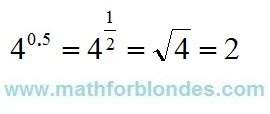4 to the power zero five

4 to the power zero five is the same as 4 to the one-second power. Four to the power of one second is the square root of 4. Taking the square root of four gives two.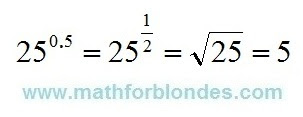25 to the power zero five

After considering the two previous examples, it will be very easy to calculate 25 to the power zero five. As real shamans, we turn zero five into one second, extract the square root of 25 and get the desired result - the root of twenty-five is five.

Why all these dances of shamans with tambourines? There are two sides to this math medal. Front side - mathematicians teach us to use mathematics. The other side of the coin is that if mathematicians simply and clearly express their thoughts in the language of mathematics, then they will not seem so smart to us, and we ourselves will not look such fools.

## 10.16.2021

### 101 cubed. Bad example.

How to find 101 cubed using abbreviated multiplication formulas? I have already shown how, using the cubes of the sum formula, one can calculate one hundred and one in a cube. There I presented one number 101 as the sum of two numbers 100 and 1. The use of simpler (more convenient in calculations) numbers makes the calculation easier.

On the Internet, I saw an example of using other formulas, the sum of cubes and the difference of cubes. This is a bad example. To find 101 cubed, use the numbers 101 and 100. This is how it looks.101 cubed. Bad example.

Why is this a bad example? I'll show you now. I'll take the number 101, the cube of which we need to find, and zero.101 cubed. Removing garbage.

By using zero, I removed all the mathematical garbage from the calculations - these are four multiplications and four additions or subtractions.

## 7.25.2021

### Cube of 101

Today we will look at an example of how you can find one hundred and one cubed using the abbreviated multiplication formulas. In other words, how to raise cube of 101 using the abbreviated multiplication formula.

The following cry for help was heard in the comments:

Help please, problem (example) from the physics and mathematics school (Russia), grade 8:

101^3

I don't understand how to solve this with abbreviated multiplication formulas.

It is very easy to cube the number 101 - you need to type the number 101 on a calculator and multiply twice by the same number 101.

101^3=101*101*101=1030301

If you don't have a calculator at hand (you never know, the phone has just been stolen), then you can calculate on a piece of paper in a column (the picture will be at the end, like checking both the calculator and the formula).

## Cube of 101 We apply the cube of sum formula.

The problem statement says that you need to find the cube of 101 using the abbreviated multiplication formula. According to math teachers, everyone should know these formulas. Naive. Where to find this formula?

You can search the Internet for a cube of sum. Google to help you. You can find these formulas in a reference book on mathematics, in a textbook on mathematics, you can ask a classmate who knows the formulas for abbreviated multiplication by heart. The formula for the abbreviated multiplication we need is called cube of sum. You will see it below.

And now the answer to the most tricky question: how to get the sum of numbers from one number? It is necessary to expand this number into terms. From the point of view of mathematics, the number of terms can be any, but ... I found the abbreviated multiplication formulas for raising a sum to a cube only for two and three terms. The formula for the cube of the sum of three terms is very complicated, I wish you never to face such a thing. But the cube of the sum of the two terms looks nice. The basic principle of expansion into terms for applying abbreviated multiplication formulas is that numbers can be easily multiplied in your head without using a calculator. For the number 101, the best option would be 101 = 100 + 1. 100 and 1 numbers is easy to multiply without a calculator. Let's see what we get.Cube of 101

I don't know about you, but I couldn't do without a piece of paper. Yes, I wrote everything down in a line, not a column, but nevertheless. And in conclusion, let's check our solution by multiplying in a column on a piece of paper.Cube of 101 in a column on a piece of paper

Well, something like this. Why do you need all this? So that you know that you can multiply not only on a calculator or in a column, but sometimes you can effectively use abbreviated multiplication formulas. If, of course, you know them.

## 12.20.2020

### Plus on minus what gives?

Positive and negative numbers were invented by mathematicians. The rules for multiplying and dividing positive and negative numbers were also invented by mathematicians. We need to learn these rules in order to tell mathematicians what they want to hear from us.

It's easy to remember the rules for multiplying or dividing positive and negative numbers. If two numbers have different signs, the result will always be a minus sign. If two numbers have the same sign, the result will always be a plus. Let's consider all possible options.

What gives plus for minus? When multiplying and dividing, plus by minus gives a minus.What gives plus for minus?

What gives a minus on plus? When multiplying and dividing, we also get a minus sign as a result.What gives a minus on plus?

As you can see, all the options for multiplying or dividing positive and negative numbers have been exhausted, but the plus sign has not appeared.

What gives a minus on a minus? There will always be a plus if we do multiplication or division.What gives a minus on a minus?

What gives plus for plus? It's quite simple here. Multiplying or dividing plus by plus always gives plus.What gives plus for plus?

If everything is clear with the multiplication and division of two pluses (the result is the same plus), then with two minuses, nothing is clear.

Why does a minus and a minus make a plus?

I can assure you that, intuitively, mathematicians have correctly solved the problem of multiplying and dividing the pros and cons. They wrote down the rules in textbooks without giving us any reasons. For the correct answer to the question, we need to figure out what the plus and minus signs mean in mathematics.

One mathematics teacher told us in the classroom: "Mathematics is an exact science, if you lie twice, you get the truth". This statement was very useful to me. Once I was solving a difficult problem with a long solution. I knew exactly what the result should be. But the result was different. I have been looking for an error in the calculations for a long time, but I could not find it. Then, a few steps before the final result, I changed one number so that the result was correct. I lied twice in the calculations and got the correct result. This is very similar to the minus on minus equals plus rule, isn't it?

## 11.02.2019

### Borsch trigonometry. Addition.

I will not tell you recipes for cooking borsch, I will talk about mathematics. What is borsch? These are vegetables cooked in water according to a specific recipe. I will consider two source components (vegetable salad and water) and the final result is borsch. Geometrically, this can be thought of as a rectangle in which one side is a salad, the other side is water. The sum of these two sides is borsch. The diagonal and area of such a rectangle are mathematical concepts and are never used in recipes.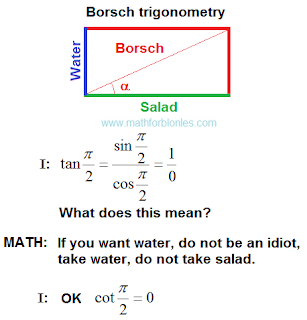Borsch trigonometry

How do salad and water turn into borsch? How does the sum turn into trigonometry? To understand this, linear angular functions will help us.

The linear angular functions

There are no linear angular functions in mathematics textbooks. But without them there can be no mathematics. Mathematical laws work whether we know them or not.

The linear angular functions are the laws of addition. This is how algebra turns into geometry, and geometry turns into trigonometry.Trigonometry addition

The main law of addition, which mathematicians do not like to talk about, requires that the summands have the same units of measurements. For salad, water and borsch it can be units of weight, volume, value or others.Law of addition

The figure shows two levels of difference. The first level is differences in the field of numbers. This is what mathematicians do. The second level is the difference in the area of units of measurement (shown in square brackets). Physicists are doing this. We can understand the third level - the differences in the field of described objects. Different objects can describe the same number of identical units of measurements. How important this is, the trigonometry of borscht will show us. Here is how linear angular functions for borsch will look.The law of addition for borsch

Part of the water and part of the salad give one serving of borsch. Now we can see what will happen when the angle of linear angular functions changes.The angle is zero

The angle is zero. We have a salad but no water. You can’t cook borsch. The amount of borsch is also zero. This does not mean that zero borsch is equal to zero water. Zero borsch can be at zero salad (right angle).The angle is greater than zero, but less than the right angle

The angle is greater than zero, but less than forty-five degrees. We have a lot of salad, but not enough water. The result is a thick borsch.

The angle is forty-five degrees. We have an equal amount of water and lettuce. Perfect borsch (forgive me the cook, it's just math).

The angle is more than forty-five degrees, but less than ninety degrees. We have a lot of water and a little salad. Get liquid borsch.Right angle

Right angle. We have water. From the salad there were only memories. We cannot cook borscht. The amount of borscht is zero. In this case, drink water.

Something like this. If you're tired of borscht, check out a few other stories.

Percentage.Percentage

Cell division.Cell division

Two friends had their shares in the general business. After killing one of them, everything went to another.The general business

The emergence of mathematics on our planet.The emergence of mathematics

All these stories in the language of mathematics are presented using linear angular functions. I will tell you separately about the real place of these functions in the structure of mathematics. In the meantime, we return to the trigonometry of borscht and consider the projections.

## 10.19.2019

### Mathematicians lie

I watched the video One minus one plus one minus one - Numberphile. Mathematicians lie. They did not perform an equality test in the course of their reasoning.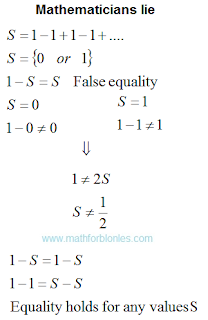Mathematicians lie

Confirmation of my reasoning about infinite sets.

If you take a closer look at all the manipulations, the error will immediately become visible. The example is written on one line and "equality" is based on the optical illusion of similarity between two different mathematical expressions. Let's write down all actions in a column, starting with the equality 1-S = S.False equality

As you can see from this example, on opposite sides of the equal sign there are a different number of terms, on the left there is one more term. Equality cannot be fulfilled if this term is not equal to zero. So the given "proof" is a common lie. I have no doubt that other "proofs" of the sum of this infinite series are also based on false statements.

## 9.29.2019

### Infinite set

Concluding the discussion of sets, you need to consider an infinite set. The fact is that the concept of "infinity" acts on mathematicians, like a boa constrictor on a rabbit. A trembling horror of infinity deprives mathematicians of common sense. Here is an example: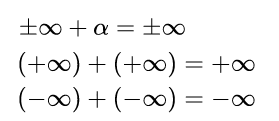Infinite set

Alpha denotes a real number. The equal sign in the above expressions indicates that if you add a number or infinity to infinity, nothing will change, the result will be the same infinity. If we take an infinite number of natural numbers as an example, then the considered examples can be represented in the following form:The set of natural numbers

To illustrate their point, mathematicians have come up with many different methods. Personally, I look at all these methods, as the dances of shamans with tambourines. In fact, they all boil down to the fact that either part of the rooms are not occupied and new guests are accommodated in them, or that some of the visitors are thrown out into the corridor to make room for guests (very humanly). I outlined my view on such decisions here in the form of a fantastic story about the Blonde. What is my reasoning based on? Relocating an infinite number of visitors requires an infinitely long time. After we vacated the first room for the guest, one of the visitors will always walk along the corridor from his room to the next one until the end of the century. Of course, the time factor can be stupidly ignored. It is mathematicians who are trying to manipulate hotel room numbers, convincing us that you can insert something that, in principle, cannot be inserted.

I will demonstrate the logic of my reasoning to you as an example of an infinite number of natural numbers. First you need to answer a very simple question: how many sets of natural numbers exist - one or many? The correct answer to this question does not exist, since we thought up the numbers ourselves; in Nature there are no numbers. Yes, Nature knows how to count, but for this she uses other mathematical tools that are not familiar to us. As Nature believes, I will tell you another time. Since we came up with numbers, we will decide how many sets of natural numbers exist. Consider both options, as befits a real scientist.

Option one. "Let us be given" one single set of natural numbers, which lies serenely on the shelf. We take this set from the shelf. Everything, there are no other natural numbers on the shelf and there is nowhere to take them. We cannot add one to this set, since we already have one. And if you really want to? No problem. We can take a unit from the set already taken by us and return it to the shelf. After that, we can take a unit from the shelf and add it to what we have left. As a result, we again get an infinite number of natural numbers. All our manipulations can be written like this: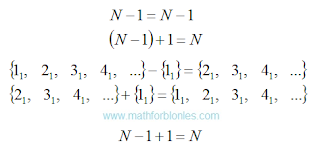One set of natural numbers

I wrote down the actions in the algebraic notation and in the notation adopted in set theory, with a detailed listing of the elements of the set. The subscript indicates that the set of positive integers is one and only. It turns out that the set of natural numbers will remain unchanged only if you subtract unit from it and add the same unit.

Option two. We have on the shelf many different infinite sets of natural numbers. I emphasize - DIFFERENT, despite the fact that they are practically indistinguishable. We take one of these sets. Then we take unit from another set of natural numbers and add to the set we have already taken. We can even add up two sets of natural numbers. Here's what we get:Many sets of natural numbers

The subscripts “one” and “two” indicate that these elements belonged to different sets. Yes, if you add one to an infinite set, the result will also be an infinite set, but it will not be the same as the original set. If we add another infinite set to one infinite set, the result is a new infinite set consisting of elements of the first two sets.

Set of natural numbers are used for counting in the same way as a ruler for measurements. Now imagine that you added one centimeter to a ruler. This will be a different a ruler, not equal to the original.

You can accept or not accept my reasoning - this is your personal matter. But if you ever run into mathematical problems, think about whether you are following the path of false reasoning, trodden by generations of mathematicians. After all, classes in mathematics, first of all, form a stable stereotype of thinking in us, and only then they add mental abilities to us (or vice versa, deprive us of free thinking).